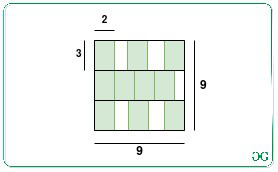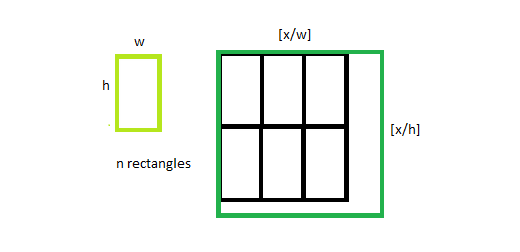Open In App

# Size of smallest square that contains N non-overlapping rectangles of given dimensions

Given two positive integers W and H and N rectangles of dimension W*H, the task is to find the smallest size of the square required such that all the N rectangles can be packed without overlapping.

Examples:

Input: N = 10, W = 2, H = 3
Output: 9
Explanation:
The smallest size of the square is 9 units to pack the given 10 rectangles of size 2*3 as illustrated in the below image:Input: N = 1, W = 3, H = 3
Output: 3

Approach: The given problem is based on the following observations:

• It can be shown that one of the optimal spacing of rectangles within a square is given by:• The maximum number of rectangles of dimension W*H, that can be fitted in the square with sides X is given by ?X/W???X/H?.
• The above function is monotonically increasing. Therefore, the idea is to use the Binary Search to find the smallest side of a square that satisfies the given condition.

Follow the steps below to solve the problem:

• Initialize two variables, say low as 1, and high as W*H*N.
• Iterate until i is less than j and perform the following steps:
• Find the value of mid as (i + j)/2.
• Now, if the value (mid/W)*(mid/H) is at most N, then update the value of high as mid.
• Otherwise, update the value of low as (mid + 1).
• After completing the above steps, print the value of high as the resultant value.

Below is the implementation of the above approach:

## C++

 `// CPP program for the above approach``#include``using` `namespace` `std;` `// Function to check if side of square X``// can pack all the N rectangles or not``bool` `bound(``int` `w, ``int` `h, ``int` `N, ``int` `x)``{``    ` `    ``// Find the number of rectangle``    ``// it can pack``    ``int` `val = (x / w) * (x / h);` `    ``// If val is atleast N,``    ``// then return true``    ``if` `(val >= N)``        ``return` `true``;` `    ``// Otherwise, return false``    ``else``        ``return` `false``;``}` `// Function to find the size of the``// smallest square that can contain``// N rectangles of dimensions W * H``int` `FindSquare(``int` `N, ``int` `W, ``int` `H)``{``    ` `    ``// Stores the lower bound``    ``int` `i = 1;` `    ``// Stores the upper bound``    ``int` `j = W * H * N;` `    ``// Iterate until i is less than j``    ``while` `(i < j)``    ``{``        ` `        ``// Calculate the mid value``        ``int` `mid = i + (j - i) / 2;` `        ``// If the current size of square``        ``// cam contain N rectangles``        ``if` `(bound(W, H, N, mid))``            ``j = mid;` `        ``// Otherwise, update i``        ``else``            ``i = mid + 1;``    ``}` `    ``// Return the minimum size of the``    ``// square required``    ``return` `j;``}` `// Driver code``int` `main()``{``    ``int` `W = 2;``    ``int` `H = 3;``    ``int` `N = 10;` `    ``// Function Call``    ``cout << FindSquare(N, W, H);``}` `// This code is contributed by ipg2016107.`

## Java

 `// Java program for the above approach``class` `GFG{``    ` `// Function to check if side of square X``// can pack all the N rectangles or not``static` `boolean` `bound(``int` `w, ``int` `h, ``int` `N, ``int` `x)``{``    ` `    ``// Find the number of rectangle``    ``// it can pack``    ``int` `val = (x / w) * (x / h);` `    ``// If val is atleast N,``    ``// then return true``    ``if` `(val >= N)``        ``return` `true``;` `    ``// Otherwise, return false``    ``else``        ``return` `false``;``}` `// Function to find the size of the``// smallest square that can contain``// N rectangles of dimensions W * H``static` `int` `FindSquare(``int` `N, ``int` `W, ``int` `H)``{``    ` `    ``// Stores the lower bound``    ``int` `i = ``1``;` `    ``// Stores the upper bound``    ``int` `j = W * H * N;` `    ``// Iterate until i is less than j``    ``while` `(i < j)``    ``{``        ` `        ``// Calculate the mid value``        ``int` `mid = i + (j - i) / ``2``;` `        ``// If the current size of square``        ``// cam contain N rectangles``        ``if` `(bound(W, H, N, mid))``            ``j = mid;` `        ``// Otherwise, update i``        ``else``            ``i = mid + ``1``;``    ``}` `    ``// Return the minimum size of the``    ``// square required``    ``return` `j;``}` `// Driver code``public` `static` `void` `main(String[] args)``{``    ``int` `W = ``2``;``    ``int` `H = ``3``;``    ``int` `N = ``10``;` `    ``// Function Call``    ``System.out.print(FindSquare(N, W, H));``}``}` `// This code is contributed by sk944795`

## Python3

 `# Python program for the above approach` `# Function to check if side of square X``# can pack all the N rectangles or not``def` `bound(w, h, N, x):` `    ``# Find the number of rectangle``    ``# it can pack``    ``val ``=` `(x``/``/``w)``*``(x``/``/``h)``    ` `    ``# If val is atleast N,``    ``# then return true``    ``if``(val >``=` `N):``        ``return` `True``      ` `    ``# Otherwise, return false``    ``else``:``        ``return` `False` `# Function to find the size of the``# smallest square that can contain``# N rectangles of dimensions W * H``def` `FindSquare(N, W, H):` `    ``# Stores the lower bound``    ``i ``=` `1``    ` `    ``# Stores the upper bound``    ``j ``=` `W ``*` `H``*``N``    ` `    ``# Iterate until i is less than j``    ``while``(i < j):``      ` `        ``# Calculate the mid value``        ``mid ``=` `i ``+` `(j ``-` `i)``/``/``2` `        ``# If the current size of square``        ``# cam contain N rectangles``        ``if``(bound(W, H, N, mid)):``            ``j ``=` `mid``        ` `        ``# Otherwise, update i``        ``else``:``            ``i ``=` `mid ``+` `1` `    ``# Return the minimum size of the``    ``# square required``    ``return` `j` `# Driver Code` `W ``=` `2``H ``=` `3``N ``=` `10` `# Function Call``print``(FindSquare(N, W, H))`

## C#

 `// C# program for the above approach``using` `System;` `class` `GFG{` `// Function to check if side of square X``// can pack all the N rectangles or not``static` `bool` `bound(``int` `w, ``int` `h, ``int` `N, ``int` `x)``{``    ` `    ``// Find the number of rectangle``    ``// it can pack``    ``int` `val = (x / w) * (x / h);` `    ``// If val is atleast N,``    ``// then return true``    ``if` `(val >= N)``        ``return` `true``;` `    ``// Otherwise, return false``    ``else``        ``return` `false``;``}` `// Function to find the size of the``// smallest square that can contain``// N rectangles of dimensions W * H``static` `int` `FindSquare(``int` `N, ``int` `W, ``int` `H)``{` `    ``// Stores the lower bound``    ``int` `i = 1;` `    ``// Stores the upper bound``    ``int` `j = W * H * N;` `    ``// Iterate until i is less than j``    ``while` `(i < j)``    ``{``        ` `        ``// Calculate the mid value``        ``int` `mid = i + (j - i) / 2;` `        ``// If the current size of square``        ``// cam contain N rectangles``        ``if` `(bound(W, H, N, mid))``            ``j = mid;` `        ``// Otherwise, update i``        ``else``            ``i = mid + 1;``    ``}``    ` `    ``// Return the minimum size of the``    ``// square required``    ``return` `j;``}` `// Driver Code``public` `static` `void` `Main()``{``    ``int` `W = 2;``    ``int` `H = 3;``    ``int` `N = 10;` `    ``// Function Call``    ``Console.WriteLine(FindSquare(N, W, H));``}``}` `// This code is contributed by ukasp`

## Javascript

 ``

Output:

`9`

Time Complexity: O(log(W*H))
Auxiliary Space: O(1)